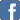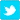# Probability: Why Is A Square Also A Rectangle

Posted on the 02 December 2020 by Rahulthepcl

To know why a square is also called a rectangle, we have to first understand the definition of both the square and rectangle. A square is a parallelogram, whose sides intersect at a 90-degree angle, and a rectangle is also a parallelogram, whose sides intersect at a 90-degree angle. In a rectangle, if the opposite sides of both the pairs are of equal length, then the rectangle can also be called a square. This means we can say that a square is also a rectangle in some cases and a square is indeed a rectangle. The other reasons why a square is also a rectangle are:• Squares and rectangles are both types of a quadrilateral, and the four interior angles are equal to 90 degrees in both square and rectangle.
• Also, the opposite sides are parallel to each other in the case of squares and rectangles.
So, it is clear that a square is a rectangle, but a rectangle is not a square. All four sides of a square are equal in length, and all four angles in a square are right angles. This is why we can say that a square is also a rectangle. But, in the case of a rectangle, the two horizontal sides are longer than the vertical sides. That means, in a rectangle, the two opposite pairs are parallel. Unlike a square in a rectangle, all four sides are not equal. So, a rectangle is not a square at all.

To understand this concept more elaborately, let's find out the characteristics of the square and rectangle:-

## Characteristics Of A Square

A square is a four-sided figure where all the sides are equal. And same as a rectangle, all the sides in a square also meet at a 90-degree angle. The other characteristics of a square include:

## Characteristics Of A Rectangle

A rectangle is a four-sided two-dimensional figure. In a rectangle, there are four straight lines, and these straight lines make a 90-degree angle. The other characteristics of a rectangle are:-

Since we have now understood the concept of a square and rectangle more elaborately, we can get an idea about why a square is also called a rectangle. Because of some of the similarities between a square and a rectangle, we can call a square a rectangle. But you always have to remember one thing that a rectangle is not a square. The reasons why a rectangle is not a square are:

All four angles of a rectangle are 90 degrees, but it is not a square because, in a rectangle, the two horizontal sides are longer than the vertical sides. That means in a rectangle. The two opposite pairs are parallel. But in the case of a square, all four sides are equal and parallel. This is the fundamental difference between a rectangle and a square, and that is why a rectangle is not a square at all.

Squares and rectangles are important topics in Geometry. These are the basic concepts of shapes that are necessary for you to learn at your school level. Along with squares and rectangles, there are also some other shapes in Geometry, such as a cube, cylinder, rhombus, cone, parallelogram, and many more. All these shapes fall under two-dimensional shapes and three-dimensional shapes categories. The characteristics and properties of all these shapes are different.

In this article, we give you a very clear idea about the concept of squares and rectangles. Now, you may be able to know why a square is also a rectangle and why a rectangle is not a square. What are the characteristics and differences between a square and a rectangle? To learn such types of similar topics online, you can enroll your kids in Cuemath. During this pandemic, every parent wants to offer the best online education to their kids and the best practice on mathematics for younger kids. Cuemath is the best and trusted educational website.

Your kids can Learn Measurement from this educational portal with the help of experienced mathematics teachers. In Cuemath, we always conduct classes for the kids by following very easy and simple methods. Our teachers try innovative ways to engage every kid in the mathematics class. To enroll your kid in such an organized virtual class, you can book a free trial class. Visit the Cuemath website and let your kids experience the best online education in mathematics.